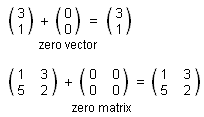Question: What is Zero ? Answer: Zero is symbolized by 0 and has several meanings. The number which does not change anything when you add it to another number. On the number line it lies mid way between -1 and + 1. It is the value of a set which has no members. The symbol for zero is 0. It is the starting point for many scales, such as temperature in degrees Celsius, where it corresponds to the freezing point of water. It is a place holder in our number system enabling us, for example, to distinguish between 4, 40, and 0.0004 The diagonals bisect the interior angles and are lines of symmetry. The number which we can add to anything without changing it (i.e the additive identity). The number which is unchanged by multiplication by other numbers. Zero is both real and rational. It is the name given to the identity element under addition for numbers, vectors and matrices. For example,